Question

# 2-The 20 foot long cantilever beam shown in the figure is subjected to the loading shown...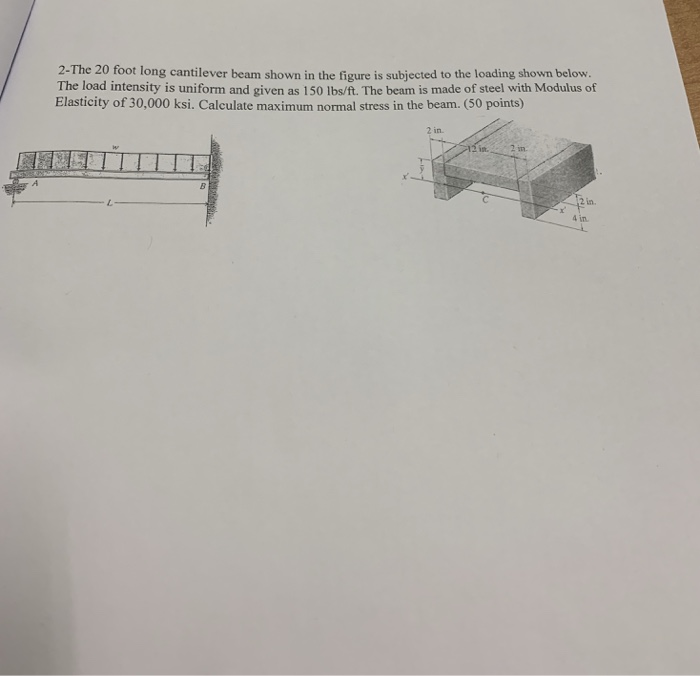2-The 20 foot long cantilever beam shown in the figure is subjected to the loading shown below. The load intensity is uniform and given as 150 lhe/ft. The beam is made of steel with Modulus of Elasticity of 30,000 ksi. Calculate maximum normal stress in the beam. (50 points) in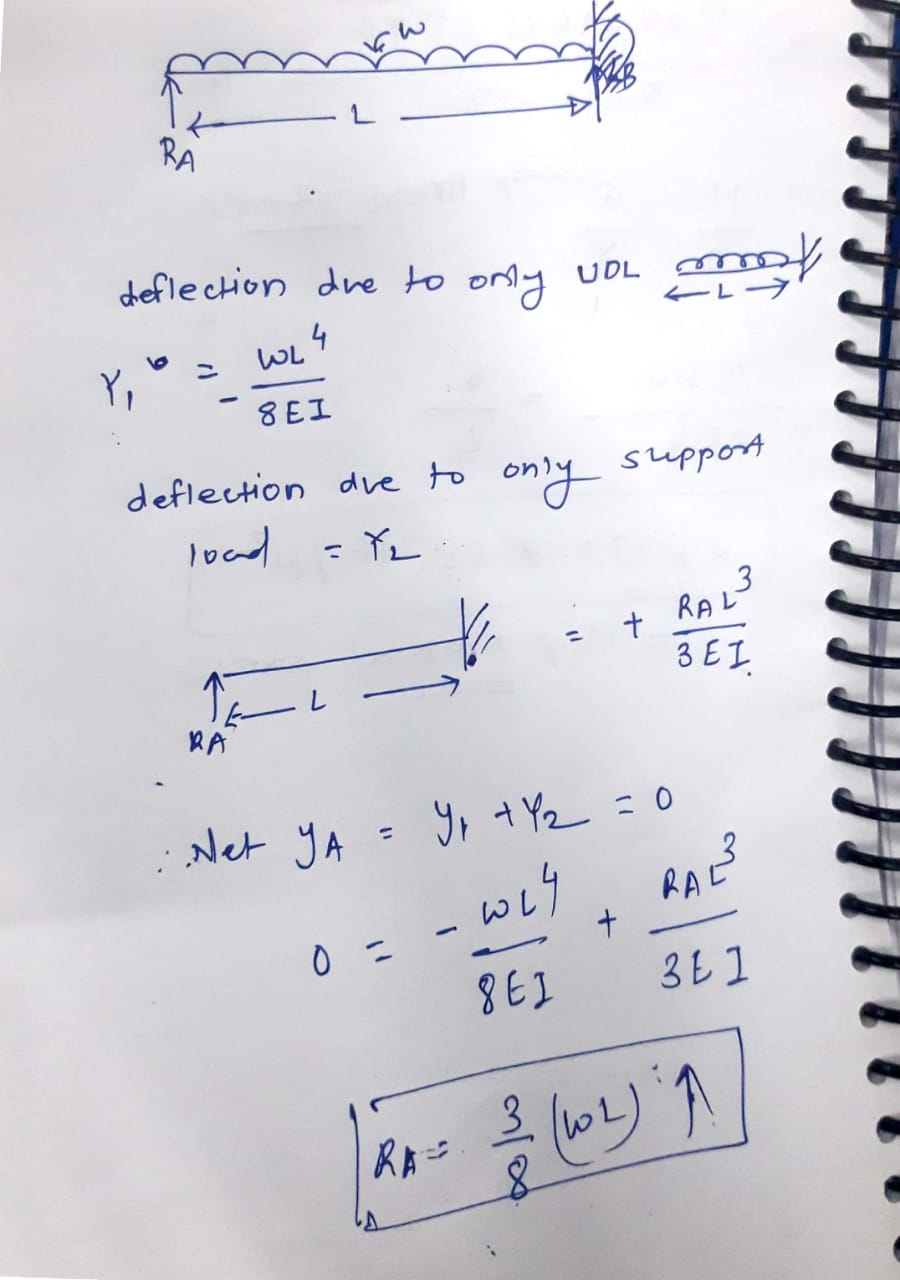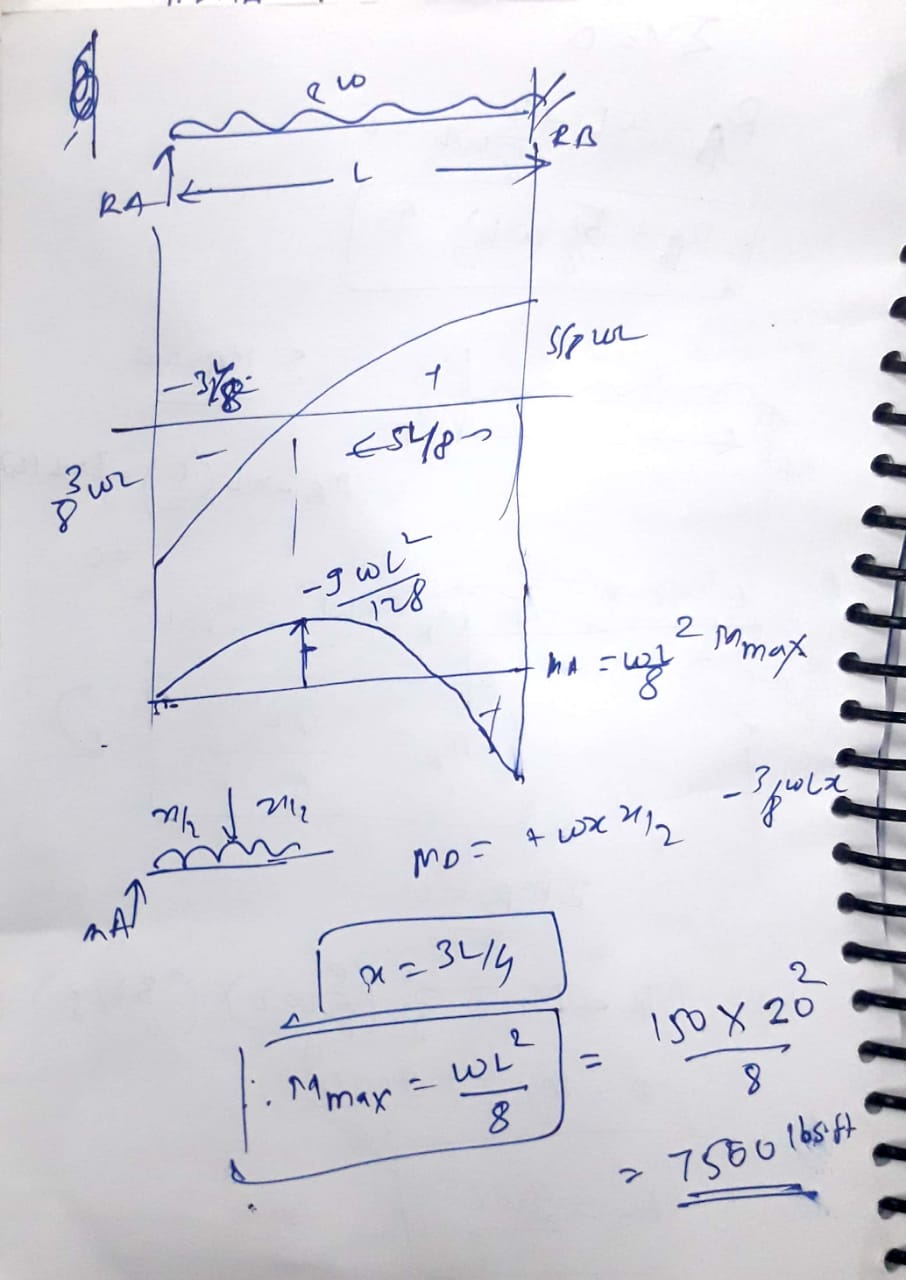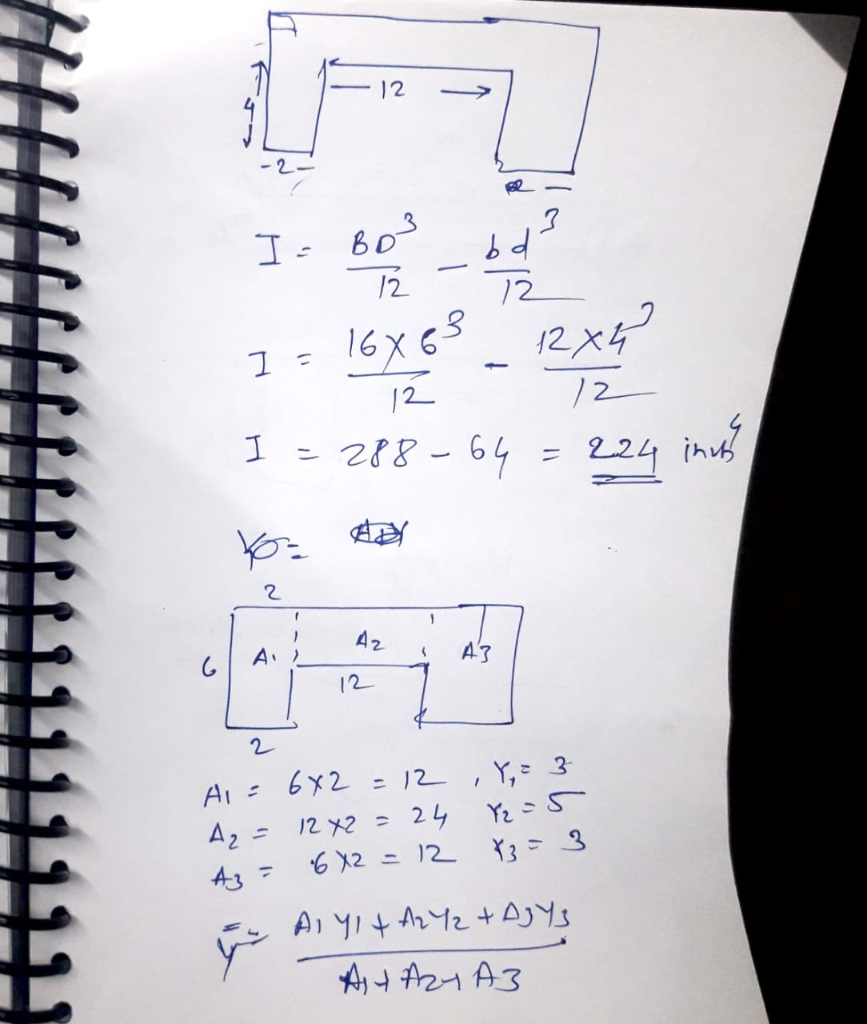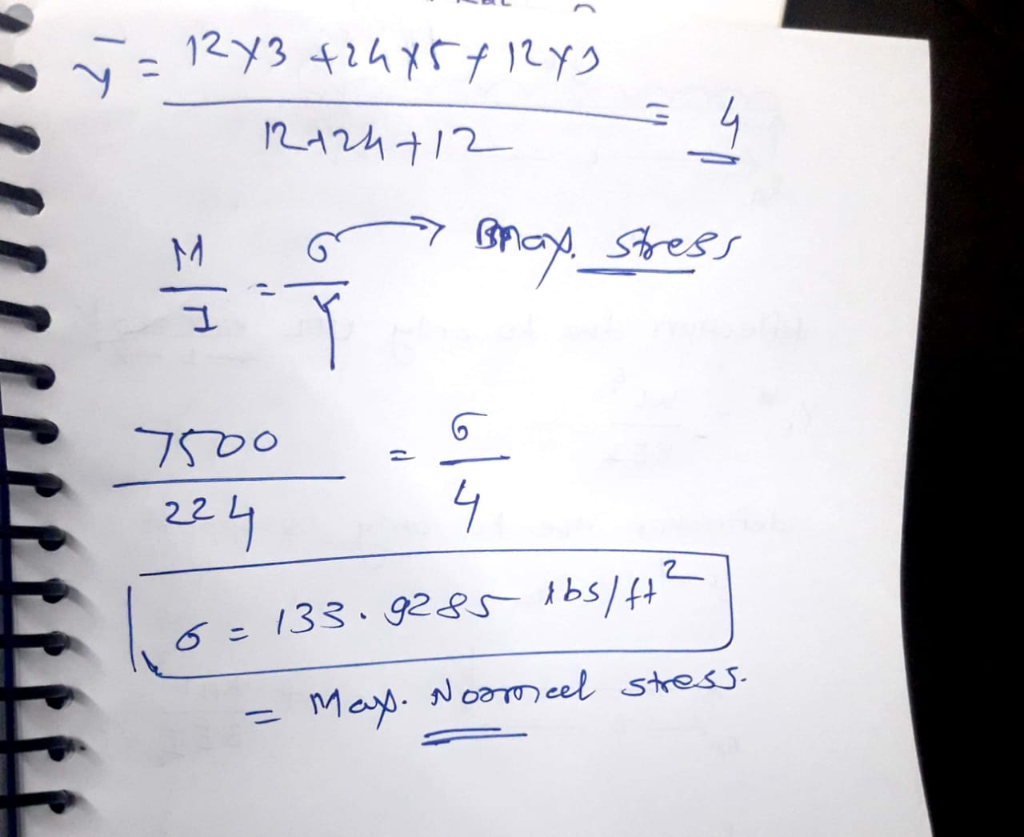#### Earn Coins

Coins can be redeemed for fabulous gifts.

Similar Homework Help Questions
• ### The figure shown below is the cross section of a steel rectangular beam. The beam is subjected to transverse loading ap...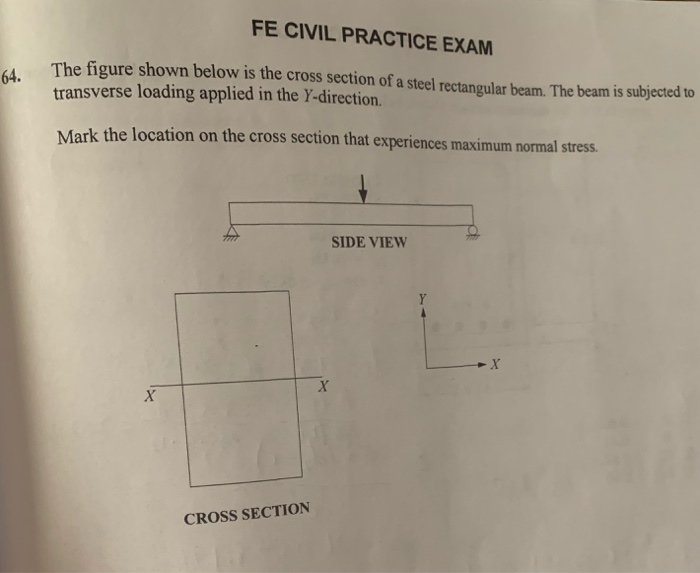The figure shown below is the cross section of a steel rectangular beam. The beam is subjected to transverse loading applied in the Y-direction. Mark the location on the cross section that experiences maximum normal stress. FE CIVIL PRACTICE EXAM The figure shown below is the cross section of a steel rectangular beam. The beam is subjected to 64. transverse loading applied in the Y-direction Mark the location on the cross section that experiences maximum normal stress SIDE VIEW CROSS...

• ### Q5. The cantilever beam, AC, is subjected to the load case shown in Figure 5. For the loading shown, do the following: [10 Marks] a) Calculate the magnitude and direction of the reactions at A b) Usi...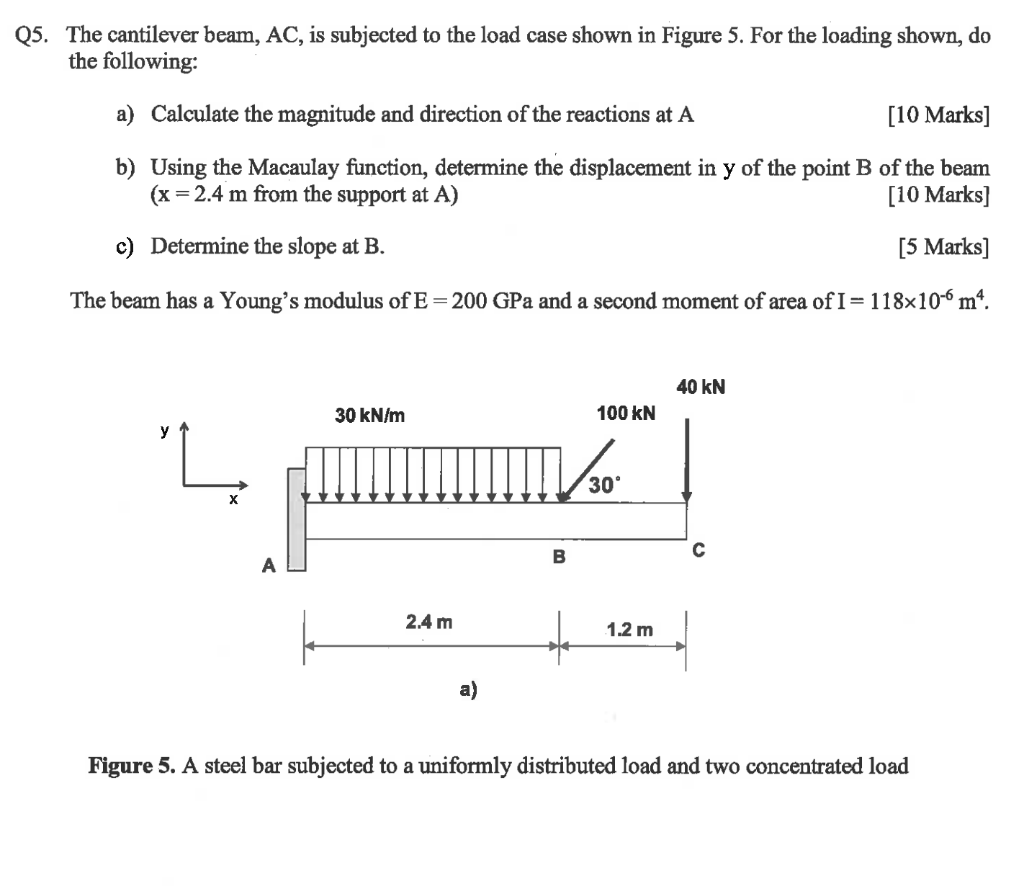Q5. The cantilever beam, AC, is subjected to the load case shown in Figure 5. For the loading shown, do the following: [10 Marks] a) Calculate the magnitude and direction of the reactions at A b) Using the Macaulay function, determine the displacement in y of the point B of the beam (x 2.4 m from the support at A) [10 Marks] c) Determine the slope at B. [5 Marks] The beam has a Young's modulus of E-200 GPa and...

• ### A beam with a square tubular cross section is subjected to the loading shown.   The cross...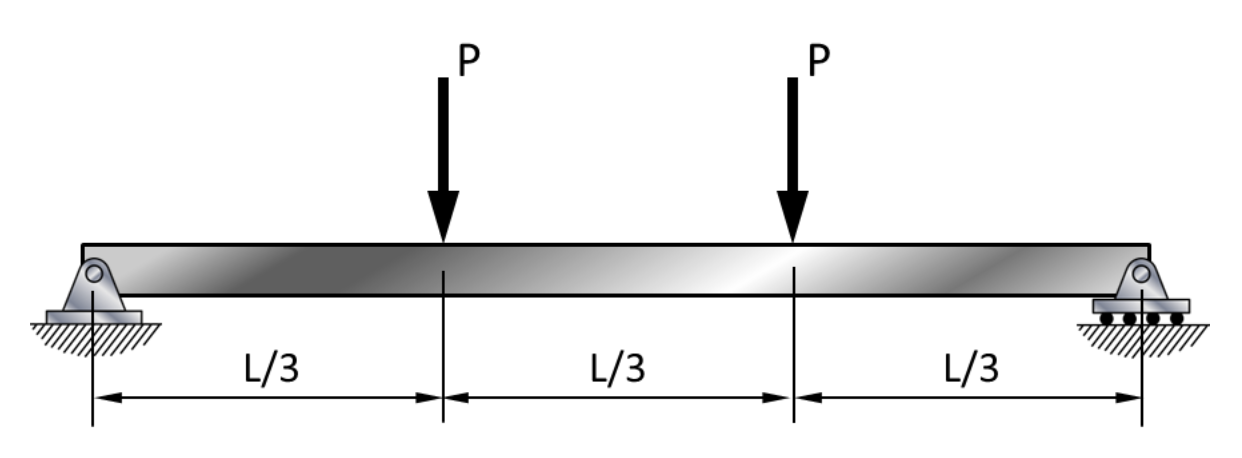A beam with a square tubular cross section is subjected to the loading shown.   The cross section of the beam is also shown. Determine the maximum bending stress in the beam given: L = 9 ft P = 1,300 lb do = 6 in di = 5.4 in 1/3 We were unable to transcribe this imageA beam with a square tubular cross section is subjected to the loading shown. The cross section of the beam is also shown. Determine the...

• ### 7 A simply supported composite beam 12 ft. long is loaded by a force of 6...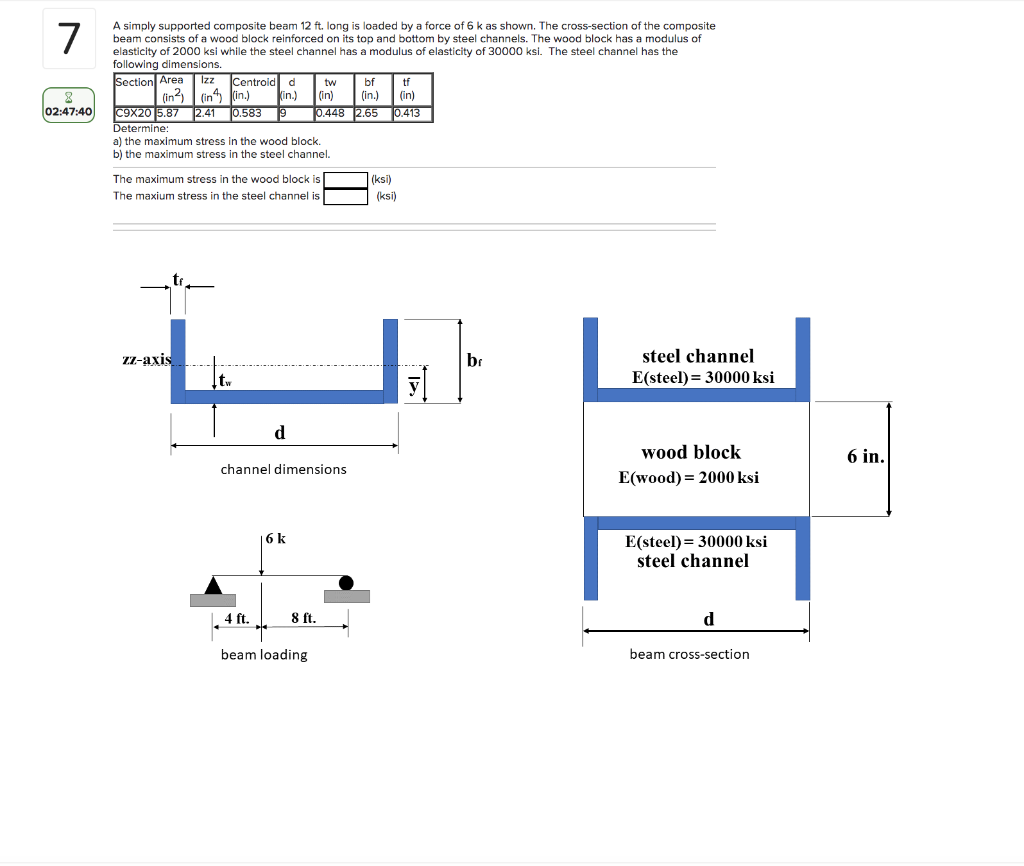7 A simply supported composite beam 12 ft. long is loaded by a force of 6 k as shown. The cross-section of the composite beam consists of a wood block reinforced on its top and bottom by steel channels. The wood block has a modulus of elasticity of 2000 ksi while the steel channel has a modulus of elasticity of 30000 ksi. The steel channel has the following dimensions. Section Area Izz Centroid d tw bf in2in in.) Kin.) (in)...

• ### If a cantilever beam is subjected to the following loading, and a cross section of the beam is provided.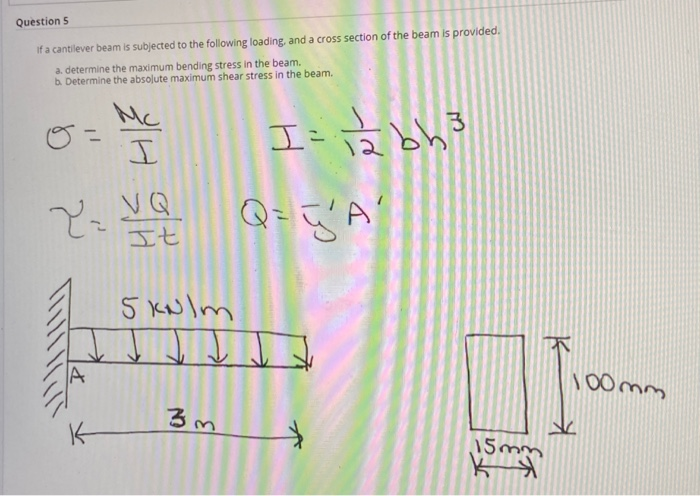If a cantilever beam is subjected to the following loading, and a cross section of the beam is provided. a. determine the maximum bending stress in the beam. b Determine the absolute maximum shear stress in the beam.

• ### A simply supported composite beam 12 ft. long is loaded by a force of 6 k...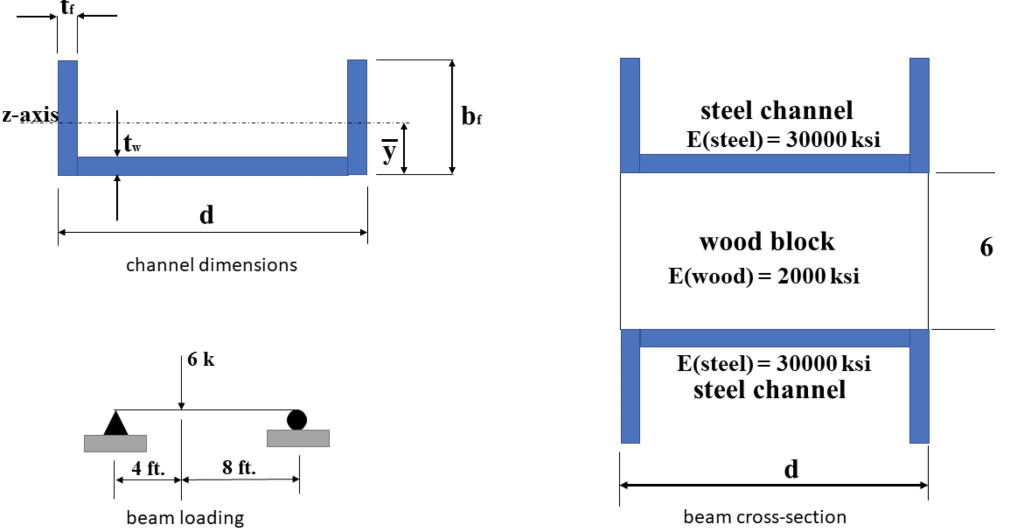A simply supported composite beam 12 ft. long is loaded by a force of 6 k as shown. The cross-section of the composite beam consists of a wood block reinforced on its top and bottom by steel channels. The wood block has a modulus of elasticity of 2000 ksi while the steel channel has a modulus of elasticity of 30000 ksi. The steel channel has the following dimensions. Section Area (in2) Izz (in4) Centroid (in.)    d (in.)    tw...

• ### Problem 1 The composite shaft, consisting of aluminum, copper, and steel sections, is subjected to the loading shown. Determine the displacement of end A with respect to end D and the normal stress i...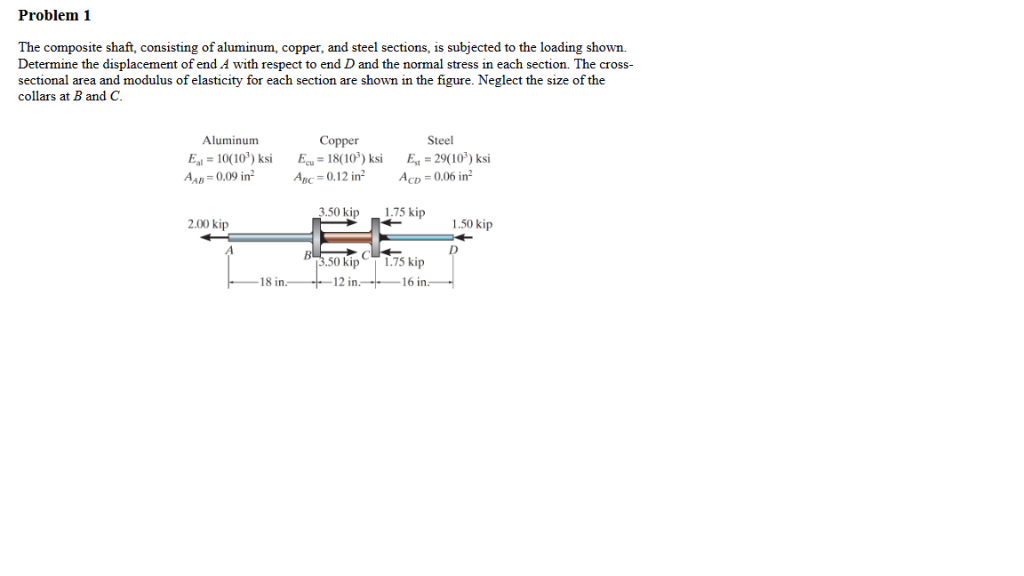Problem 1 The composite shaft, consisting of aluminum, copper, and steel sections, is subjected to the loading shown. Determine the displacement of end A with respect to end D and the normal stress in each section. The cross- sectional area and modulus of elasticity for each section are shown in the figure. Neglect the size of the collars at B and C. Aluminum Copper 18(00)ksi E-29(10') ksi Steel -1010') ksi AB-0,09 in AcD 0.06 in Авс 0.1 2 i 2...

• ### The cantilever beam is subjected to a concentrated load of P = 29 kips. The cross-sectional dimen...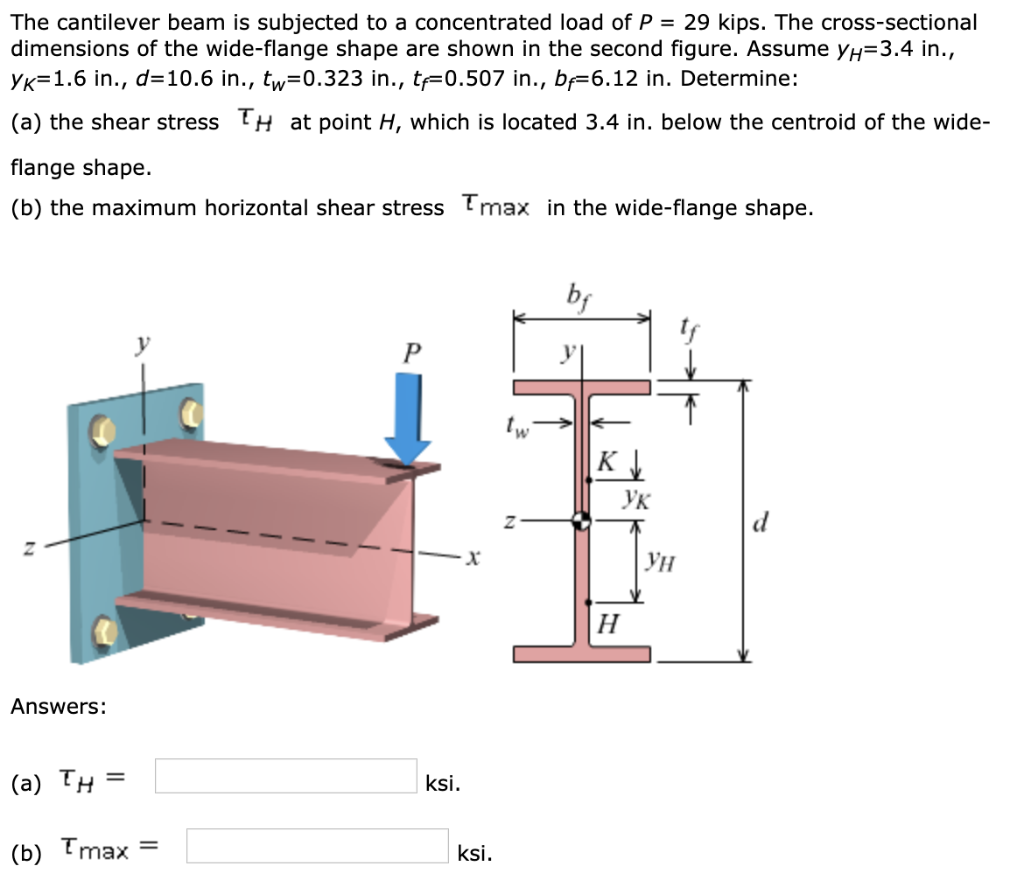The cantilever beam is subjected to a concentrated load of P = 29 kips. The cross-sectional dimensions of the wide-flange shape are shown in the second figure. Assume yH=3.4 in., yK=1.6 in., d=10.6 in., tw=0.323 in., tf=0.507 in., bf=6.12 in. Determine: The cantilever beam is subjected to a concentrated load of P 29 kips. The cross-sectional dimensions of the wide-flange shape are shown in the second figure. Assume y,-3.4 in., Ук_ 1.6 in., d-10.6 in., t,-0.323 in., tf-0.507 in., bf-6.12...

• ### 14-1. For the beam shown in the figure, a) b) c) d) Find the magnitude and...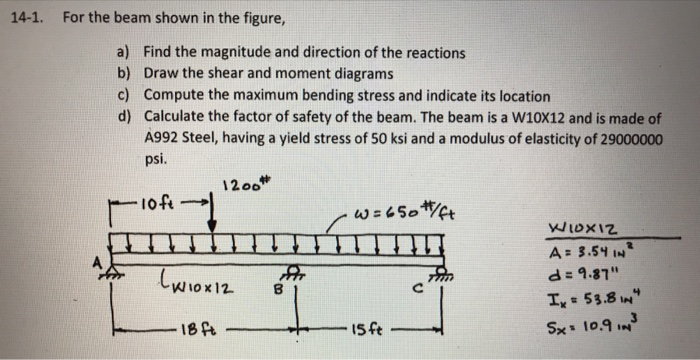14-1. For the beam shown in the figure, a) b) c) d) Find the magnitude and direction of the reactions Draw the shear and moment diagrams Compute the maximum bending stress and indicate its location Calculate the factor of safety of the beam. The beam is a W10x12 and is made of A992 Steel, having a yield stress of 50 ksi and a modulus of elasticity of 29000000 psi. 12oo A 3.5IN d = 9.87" Ix 53.8 IN

• ### The W10 × 15 cantilevered beam is made of A-36 steel and is subjected to the loading shown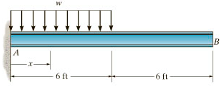The W10 × 15 cantilevered beam is made of A-36 steel and is subjected to the loading shown. Suppose that w=2.5 kip/ft. E = 29(103) ksi and I = 68.9 in4.(Figure 1) Part A Determine the slope of the beam B, measured counterclockwise from the positive x axis. Express your answer using three significant figures. Part B Determine the displacement of the beam at B.